﻿ 高强螺栓钢管柱端板节点在循环荷载作用下的F-δ曲线函数模型研究 Performance Investigation on F-δ Curve Function Model of End Plate Connection with High-Strength Bolts under Cyclic Loading

Hans Journal of Civil Engineering
Vol.07 No.03(2018), Article ID:25132,10 pages
10.12677/HJCE.2018.73054

Performance Investigation on F-δ Curve Function Model of End Plate Connection with High-Strength Bolts under Cyclic Loading

Xiaolei Li, Zhiyu Wang

Institute of Architecture and Environment, Sichuan University, Chengdu Sichuan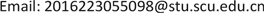Received: May 10th, 2018; accepted: May 22nd, 2018; published: May 29th, 2018ABSTRACT

In order to establish the F-δ curve function model of end plate connection with high-strength bolts under cyclic loading, four conventional function models were used to compute based on measured data and the fitting degree of all function curves was analyzed. The results showed that the polynomial function model can fit the actual curve better and the logarithmic function model shows its performance is more effective than other function models at elastoplastic stage.

Keywords:End Plate Connection with High-Strength Bolts, F-δ Curve, Polynomial Function Model, Logarithmic Function Model1. 引言

2. 常见M-θ曲线模型函数

2.1. 线性函数模型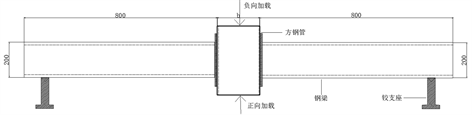Figure 1. Test set-up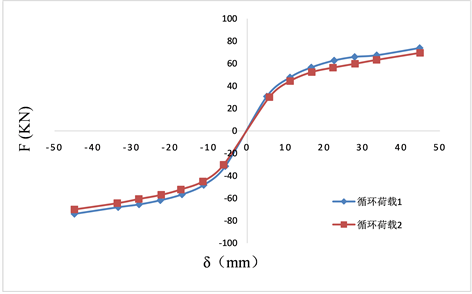(a) 试件G-8-6-15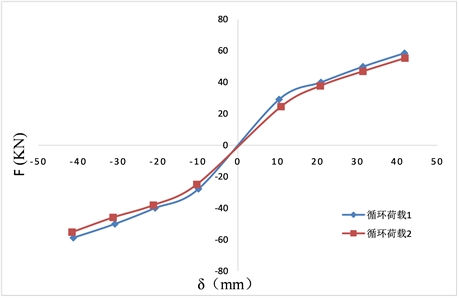(b) 试件G-8-6-20(M12)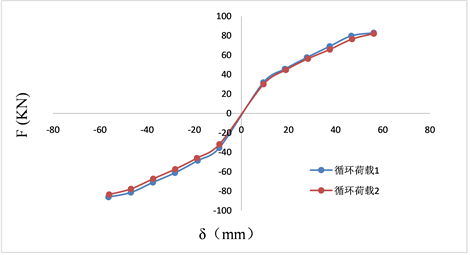(c) 试件G-8-6-20(M16)

Figure 2. Load displacement curve of all specimens

2.2. 多项式函数模型

$\theta ={C}_{1}{\left(KM\right)}^{1}+{C}_{2}{\left(KM\right)}^{3}+{C}_{3}{\left(KM\right)}^{5}$ (1)

2.3. 幂函数模型

1) 二参数幂函数模型  ：

$\theta ={C}_{1}{M}^{{C}_{2}}$ (2)

2) 三参数幂函数模型  ：

$\theta =\frac{M}{{K}_{t}}\frac{1}{\left[1-{\left(M/{M}_{u}\right)}^{n}\right]}$ (3)

2.4. 指数函数模型

1) 三参数指数函数模型：

$\frac{M}{{M}_{U}}=n\left[\mathrm{ln}\left(1+\frac{\theta }{n{\theta }_{0}}\right)\right]$ (4)

2) 四参数指数函数模型：

Yoke Leong Yee和Robert E  共同提出了该函数模型，他们研究发现之前提出的指数函数模型表达式多用回归分析得到，并不具备实际的物理意义，因此在试验数据的基础上，用数学方法建立了一种更为全面的指数函数模型，其表达式如下：

$M={M}_{u}\left\{1-\mathrm{exp}\left[\frac{-\left({K}_{i}-{K}_{p}+C\theta \right)\theta }{{M}_{u}}\right]\right\}+{K}_{p}\theta$ (5)

1) 当θ = 0时，M = 0，即曲线通过原点；

2) 当θ = 0时， $\frac{\text{d}M}{\text{d}\theta }={K}_{i}$ ，即曲线在原点的斜率等于节点的初始刚度；

3) 当θ → ∞时， $\frac{\text{d}M}{\text{d}\theta }={K}_{p}$ ，即当θ趋近于无穷大时，曲线斜率等于节点应变强度；

4) 对于任何θ值而言，曲线上该店的斜率都对应相应的节点刚度；

5) 函数模型中的参数均具有明确的物理意义；

6) 函数模型中的参数能够被简单确定，减小了换算过程中误差的产生，使得模拟的曲线更贴近真实情况；

7) 函数模型的表达式简单，便于计算。

3. 曲线的试验研究

3.1. 多项式函数

$F={C}_{1}{\delta }^{2}+{C}_{2}\delta +{C}_{3}$ (6)

1) 螺栓规格是试件G-8-6-20(M12)和G-8-6-20(M16)之间的唯一变量，前者螺栓规格为M12，后者螺栓规格是M16，从二者系数表可以看出，当提高螺栓规格，系数C1、C2和C3值均增加，其中C3增加更突出。

2) 钢管柱截面面积是试件G-8-6-25和G-8-6-15之间的唯一变量，前者为钢管柱截面面积是250 × 250 mm，后者钢管柱截面面积是150 × 150 mm，从二者系数表可知，当钢管柱截面面积减小，系数C1的值减小，C2和C3值增加。

3.2. 指数函数

$F={C}_{1}{\text{e}}^{{C}_{2}\delta }$ (7)

1) 螺栓规格与端板节点的指数函数系数有关。从试件指数函数系数表可以看出，当提高螺栓规格，系数C1的值增大，C2的值则呈现一定程度的减小；

2) 钢管柱截面面积与端板节点的指数函数系数有关。从试件指数函数系数表可知，当钢管柱截面面积减小，系数C1、C2的值均增大。

3.3. 对数函数

$F={C}_{1}\mathrm{ln}\left(\delta \right)-{C}_{2}$ (8)

1) 螺栓规格与端板节点的指数函数系数有关。从试件对数函数系数表可以看出，当提高螺栓规格，系数C1的值增大，C2的值则呈现一定程度的减小；

2) 钢管柱截面面积与端板节点的指数函数系数有关。从试件指数函数系数表可知，当钢管柱截面面积减小，系数C1、C2的值均增大。

3.4. 幂函数

$F={C}_{1}{\delta }^{{C}_{2}}$ (9)

1) 螺栓规格与端板节点的幂函数系数C1有关，与系数C2无关。从试件G-8-6-20(M12)和G-8-6-20(M16)系数表可以看出，当提高螺栓规格，系数C1的值一定程度地增大，而C2的值并无明显增大或减小的规律可循；

2) 钢管柱截面面积与端板节点的幂函数系数有关。从试件幂函数系数表可知，当钢管柱截面面积减小，系数C1和C2的值增大。

4. 函数模型曲线分析(a) 正向加载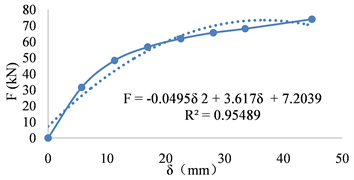(b) 负向加载

Figure 3. Polynomial function simulation curve of speciment G-8-6-15 (first cyclic loading)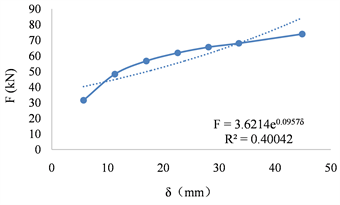(a) 正向加载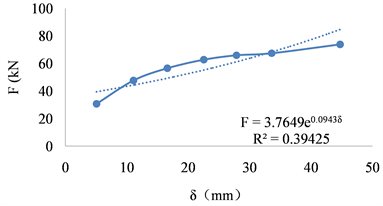(b) 负向加载

Figure 4. Exponential function simulation curve of speciment G-8-6-15 (first cyclic loading)

1) 指数函数模型和幂函数模型的函数曲线与试件实际的F-δ曲线差别极大，而多项式函数模型和对数函数模型的函数曲线更加贴近试件实际的F-δ曲线；

2) 在循环加载的作用下，高强螺栓钢管柱端板连接件钢管壁厚或螺栓会经历弹性阶段、弹塑性阶段和塑性阶段，因此实际的F-δ曲线的初始部分可以看作近似的线性关系，此时的斜率为初始刚度，而随着荷载的增加，曲线不能再看成近似线性，此时刚度为变值，故承载力极限状态设计时不能用初始刚度，这是高强螺栓钢管柱端板节点的F-δ曲线重要的特性之一，从四种函数模型的函数曲线比较来看，只有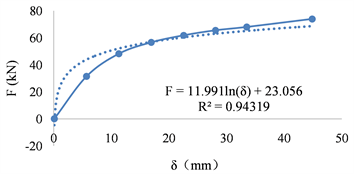(a) 正向加载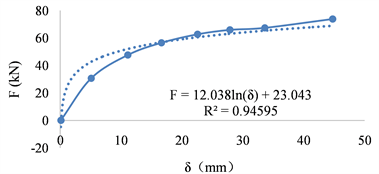(b) 负向加载

Figure 5. Logarithmic function simulation curve of speciment G-8-6-15 (first cyclic loading)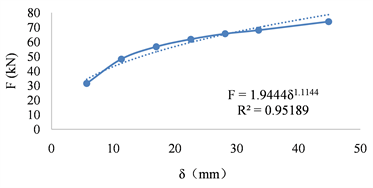(a) 正向加载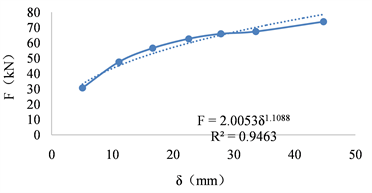(b) 负向加载

Figure 6. Power function simulation curve of speciment G-8-6-15 (first cyclic loading)

3) 四种函数模型的表达式中，只有多项式函数模型和对数函数模型的表达式较为简单，方便计算，减小了换算过程中误差的产生。

5. 结论

1) 多项式函数模型、指数函数模型、对数函数模型和幂函数模型均能在一定程度上模拟试件实际的荷载—位移曲线，当试件的模型参数发生变化时，各函数模型的无量纲系数呈现规律性的增大或减小。

2) 指数函数模型和幂函数模型计算复杂且不能模拟试件在弹性阶段发生较小位移时的荷载—位移曲线，对于需要计算初始刚度的试件模型建议不采用这两种函数模型。

3) 多项式函数模型能最大程度的还原试件实际的荷载—位移曲线，但弹塑性阶段拟合较差，采用对数函数模型可弥补这一缺陷。

Performance Investigation on F-δ Curve Function Model of End Plate Connection with High-Strength Bolts under Cyclic Loading[J]. 土木工程, 2018, 07(03): 475-484. https://doi.org/10.12677/HJCE.2018.73054

1. 1. 邢贵洋, 王升博, 周光禹. 高强螺栓端板连接节点的弯矩-转角关系研究[J]. 山西建筑, 2006, 32(7): 52-53.

2. 2. Frye, M.J. and Morris, G.A. (1975) Analysis of Flexibly Connected Steel Frames. Canadian Journal of Civil Engineering, 2, 280-291. https://doi.org/10.1139/l75-026

3. 3. Colson, A. and Louveau, J.M. (1983) Connections Incidence on the Inelastic Behavior of Steel Structures. Euromech Colloquium.

4. 4. Wu, F.H. and Chen, W.F. (1990) A Design Model for Semi-Rigid Connections. Engi-neering Structures, 12, 88-97. https://doi.org/10.1016/0141-0296(90)90013-I

5. 5. Yee, Y.L. and Melchers, R.E. (1986) Moment-Rotation Curves for Bolted Connections. Journal of Structural Engineering, 112, 615-635. https://doi.org/10.1061/(ASCE)0733-9445(1986)112:3(615)

6. 6. Frye, M.J. and Morris, G.A. (1975) Analysis of Flexibly Connected Steel Frames. Canadian Journal of Civil Engineering, 2, 280-291. https://doi.org/10.1139/l75-026